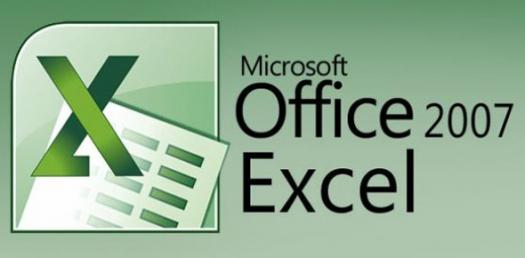# Can You Pass The Microsoft Excel 2007 Basic Test? Trivia Quiz

10 Questions | Attempts: 1771
ShareSettingsThe Microsoft Excel or MS Excel is a spreadsheet software developed by Microsoft. The core purpose of this application is to manage financial modeling, data processing using the graphing tools, pivot tables, and calculation. This quiz has been designed to test your knowledge about essential functions and tools. Read the questions carefully and answer. Let's try out the quiz. All the best!

• 1.
Why is Excel a useful program?
• A.

Who knows? Baba Tariq just told me to use it. I don't ask questions!

• B.

It helps keep data organized.

• C.

It can perform calculations.

• D.

It can easily create graphs and charts from data.

• E.

B, C and D

• 2.
If you want to make a chart, how would you get to the chart-making wizard?
• A.

Click on the drop down menu "Insert" and select "Chart."

• B.

• C.

It's impossible to make a chart

• D.

Click on the drop down menu "Tools" and select "Chart."

• 3.
True or False: Excel can calculate averages and sums.
• A.

True

• B.

False

• 4.
How many different types of charts can you make in Excel?
• A.

One - Column Chart.

• B.

Two - Column Chart and Bar Graph.

• C.

Three - Column Chart, Bar Graph, Pie Chart.

• D.

Many!!

• 5.
If you want to add a number of cells together, what is the formula you use?
• A.

=total(cells)

• B.

=sum(cells)

• C.

• D.

=halloween(cells)

• 6.
When might you use Excel in the future?
• A.

When organizing data in one of your classes for example in (science) class

• B.

When making graphs in one of your classes for example in (math) class

• C.

When trying to decide if you're late for watching a movie

• D.

A and B.

• 7.
What is the intersection of a column and a row on a worksheet called?
• A.

Column

• B.

Value

• C.

• D.

Cell

• 8.
What type of chart is useful for comparing values over categories?
• A.

Pie Chart

• B.

Column Chart

• C.

Line Chart

• D.

Dot Graph

• 9.
A feature that displays only the data in column (s) according to specified criteria
• A.

Formula

• B.

Sorting

• C.

Filtering

• D.

Pivot

• 10.
Statistical calculations and preparation of tables and graphs can be done using
• A.

• B.

Excel

• C.Back to top